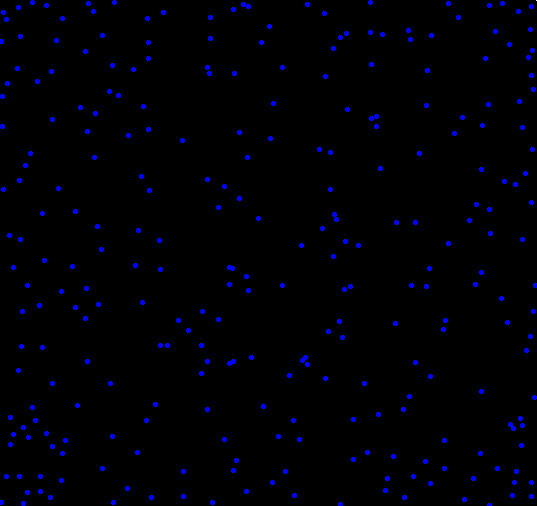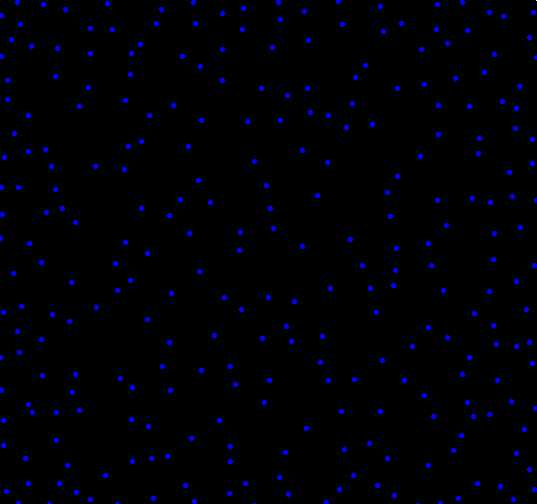[This article was first published on Probability and statistics blog » r, and kindly contributed to R-bloggers]. (You can report issue about the content on this page here)
Want to share your content on R-bloggers? click here if you have a blog, or here if you don't.

What if you wanted to randomly place objects into a field, and the more objects you had, the more they rejected newcomers placed nearby? To find out, I setup a simulation. The code, shown at the end, isn’t all that interesting, and the plots shown below aren’t all that special. I think there is one interesting part of this, and that’s how the clustering changes depending on how distance is measured. One of the plots uses the traditional “L2″ distance, the other uses L1″ (Manhattan taxi cab) measure . Each plot shown below has almost exactly the same number of dots (277 vs 279). Can you tell which uses L1 and which uses L2 just by looking?

Plot A:Plot B:Here’s the code. Run it and see for yourself. Make sure to change adjust the values which have comments next to them. Uncommenting “print(force)” can help you pack a maxRepulse value.

```calcRepulse <- function(x,y,dots,use="L2") {
force = 0
i = 1
while(i <= dim(dots) && dots[i,1] != 0) {
if(use == "L2") {
force = force + 1/( (x-dots[i,1])^2 + (y-dots[i,2])^2 )
} else if(use == "L1") {
force = force + 1/( abs(x-dots[i,1]) + abs(y-dots[i,2]) )
}
i = i+1
}
# print(force)
return(force)
}

par(bg="black")
par(mar=c(0,0,0,0))
plot(c(0,1),c(0,1),col="white",pch=".",xlim=c(0,1),ylim=c(0,1))

place = 1 #Maximum number of dots to place, change this to something bigger
dots = matrix(rep(0,place*2),ncol=2)
maxTries = place * 10
maxRepulse = 1 # Anything above this will be rejected as too repulsive a location
dist2use = "" # Pick L1 or L2

placed = 0
tries = 0

while(placed < place && tries < maxTries) {
x = runif(1)
y = runif(1)

if(calcRepulse(x,y,dots,dist2use) < maxRepulse) {
dots[(placed + 1),1] = x
dots[(placed + 1),2] = y
placed = placed + 1
points(x,y,col="blue",pch=20)
}

tries = tries + 1
}```